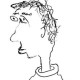In complex geometry, the most interesting class of complex manifolds is probably the Kahler class. In the non-archimedean world, say over a fixed p-adic base field$K$, the analogue of a compact complex manifold is a smooth proper rigid analytic space. In some ways, these are already surprisingly “close” to being Kahler – in particular, the Hodge-de Rham spectral sequence of such a space always degenerates at$E_1$. However, Hodge symmetry can definitely fail. A standard example is the non-archimedean Hopf surface$X = \mathbf{A}^2_{K} \smallsetminus \{ (0,0) \} / p^{\mathbf{Z}}$ where$p^n$ acts through diagonal multiplication. By a fun direct calculation, one checks that$H^0(X,\Omega^1_X)=0$ and$H^1(X,\mathcal{O}_X) = K$, so Hodge symmetry fails in degree one.

We now see a natural question: is there is some non-archimedean analogue of the Kahler condition which restores Hodge symmetry? Two years ago, Shizhang Li hit upon the following candiate condition:

A smooth proper rigid space$X$ satisfies (*) if it admits a formal model$\mathfrak{X}$ over$\mathcal{O}_K$ whose special fiber is projective (as opposed to merely proper).

Using fantastic ideas due to Shizhang, we managed to prove the following suggestive result.

Theorem. Let$X$ be a smooth proper rigid space satisfying (*). Then$h^{1,0}(X) = h^{0,1}(X)$.

Of course, one can then guess that (*) implies Hodge symmetry in all degrees. This speculation seems to have caught the imagination of others in the field, but until recently I personally regarded it as not much more than wishful thinking. However, my perspective completely changed a month ago, when I learned from Shizhang that, according to Robert Friedman, the archimedean analogue of “(*) implies Hodge symmetry” is a theorem! More precisely, we have the following result:

Theorem. Let$D$ be the complex disk, with$D^\times =D \smallsetminus \{0 \}$ the punctured disk. Let$f:Y \to D$ be a proper map of complex analytic spaces. Suppose that$f^{-1}(D^\times) \to D^\times$ is a submersion, and that the central fiber$Y_0=f^{-1}(0)$ is the analytification of a projective (and not necessarily smooth) algebraic variety. Then for all$t \in D^\times$ with$|t| \ll 1$, the fiber$Y_t$ satisfies Hodge symmetry and Hodge-de Rham degeneration.

Of course, the analogy is that$\mathfrak{X} \to \mathrm{Spf} \mathcal{O}_K$ is analogous to$Y \to D$, and$X$ is analogous to the “nearby” fibers$Y_t$ with$0<|t| \ll 1$.

The proof of this theorem uses the full power of mixed Hodge theory. In fact the claim about Hodge-de Rham degeneration is exactly Corollary 11.24 in the book of Peters-Steenbrink. Hodge symmetry is even more subtle, and the argument for this doesn’t seem to be written down anywhere; Friedman explained it to Shizhang, who explained it to me, but the details entailed such a horrible explosion of gradings, filtrations, and multi-indices that I can’t hope to reproduce it here.

Anyway, I’m now completely convinced that Shizhang’s condition (*) implies Hodge symmetry in all degrees, and that this is truly the “right” p-adic analogue of the Kahler condition.

Posted on Categories Math

## 6 thoughts on “p-adic Kahler manifolds”

1.Rahul Krishna says:

Isn’t (*) strictly stronger than Kahler for complex analytic spaces? If I recall, Voisin disproved Kodaira’s conjecture that every compact Kahler manifold can be deformed to something projective.

Still, the idea that (*) implies Hodge symmetry in the $p$-adic world is quite spicy…

Like

1.arithmetica says:

I don’t think it’s stronger: note that the nearby fibers Y_t need not be Kahler! I guess it’s neither weaker nor stronger.

Like

1.Laurent says:

Mr. Hansen, I really do not understand something here.

Theorem 9.23 (HODGE THEORY AND COMPLEX
ALGEBRAIC GEOMETRY I). Let φ : X → B be a family of complex manifolds, 0 ∈ B. If the
fibre X0 is Kahler, then so is Xb for all b sufficiently near 0.

Theorem 3 (On the homotopy types of Kahler manifolds and the
birational Kodaira problem). In any even dimension ≥ 8, there exist compact Kahler manifolds X,
such that no compact bimeromorphic model X0 of X has the homotopy type of a
projective complex manifold.

What exactly is your claim, “neither weaker nor stronger”? I do not really know anything about singularities, but for a closed Kaehler *manifold*, all nearby fibers are going to be Kaehler. Or are you claiming that while it is true that there is a small disk over which the fibers are Kaehler and there is a small disk over which the fibers satisfy Hodge symmetry, these two disks are distinct? Are you also claiming that while some closed Kaehler manifolds are not homotopic to any smooth complex projective variety, they are necessarily homotopic to a *singular* projective variety? Could you provide some references?

Like

2.Rahul Krishna says:

Ah, I see. Silly me.

Like

1.arithmetica says:

@Laurent: Yes, if f is a submersion and Y_0 is Kahler, then Y_t will also be Kahler for all small t, as you noted. But the Kahler condition doesn’t even make sense if Y_0 is singular; by definition, a Kahler manifold is smooth. Does that clear things up a bit?

Like

3.mayorliatmath says:

In the Y \to D scenario described above, after using Hironaka’s embedded resolution of singularity, we may assume that the central fiber is not only projective but also simple normal crossing. And so maybe (and only maybe) those fibres Y_t (with t sufficiently small) is Kahler (Bob actually conjectured this to be true).

Like